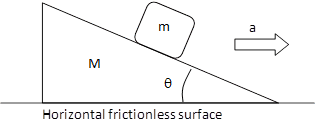# Net force on wedge and block

• PAstudent

## Homework Statement

[/B]
For the situation shown in the diagram, the block of mass m remains in place on the sloping front of the accelerating wedge, even though that sloping front is frictionless. Given these values, M = 20.0 kg, m = 4.00 kg, and theta= 34.0, calculate the net force acting on the wedge plus block (M+m) system.## Homework Equations

Newton's second law of motion

## The Attempt at a Solution

At first, I tried turning the frame of reference so my normal force for the block was on the y-axis on my free body diagram. However, when I was looking at it more, I was confused on what I was actually trying to find with the wedge.

## Homework Statement

[/B]
For the situation shown in the diagram, the block of mass m remains in place on the sloping front of the accelerating wedge, even though that sloping front is frictionless. Given these values, M = 20.0 kg, m = 4.00 kg, and theta= 34.0, calculate the net force acting on the wedge plus block (M+m) system.

View attachment 88942

## Homework Equations

Newton's second law of motion

## The Attempt at a Solution

At first, I tried turning the frame of reference so my normal force for the block was on the y-axis on my free body diagram. However, when I was looking at it more, I was confused on what I was actually trying to find with the wedge.
A vague description of an attempted method does not qualify as a posted attempt. We need to see your working.